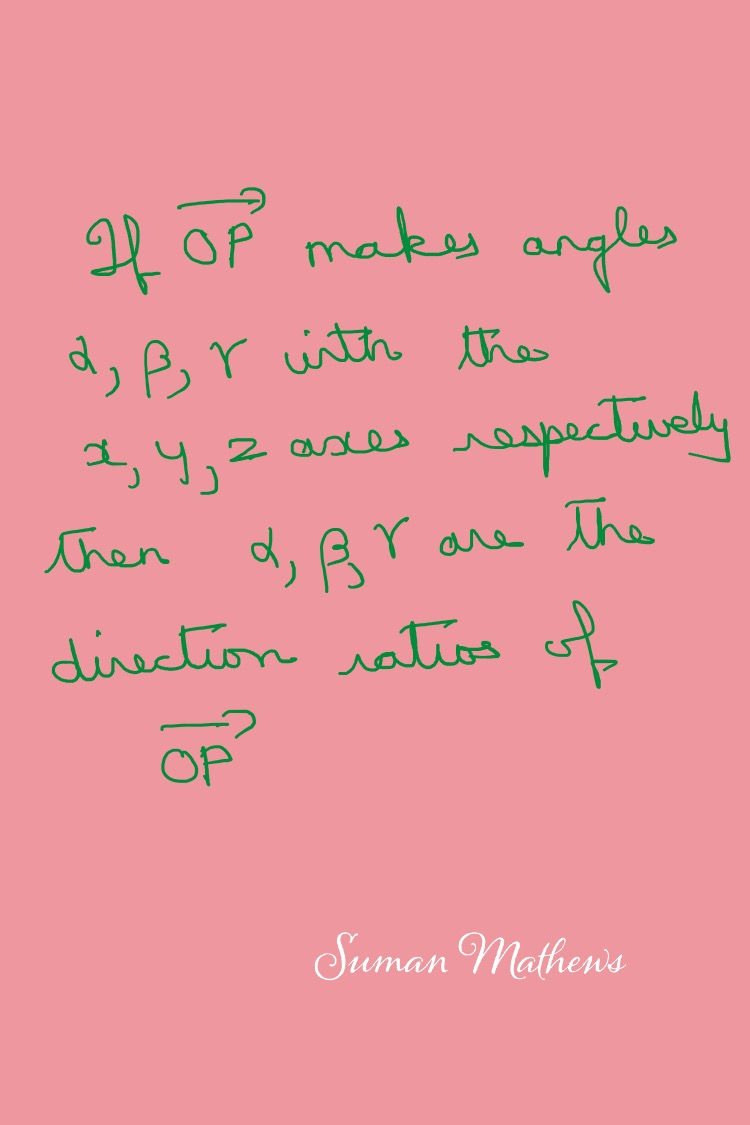top of page
Search

# How to Study Vectors for Class 12 Mathematics-NCERT, ISC,CUET

## So what is a vector? A vector is a quantity which has both magnitude and direction. The magnitude of a vector is also called its modulus and is defined as follows.## You'll need to note that while calculating vectors, it is final point- initial point.## Next, you'll proceed to learn about direction angles or direction ratios and how to compute the direction cosines given the direction ratios. An important property of direction cosines is also illustrated here.